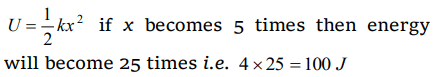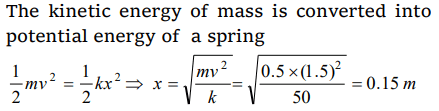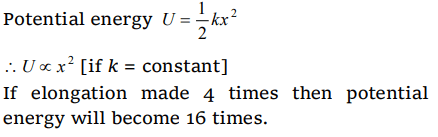## Work, Energy and Power Questions and Answers Part-6

1. A spring of force constant 800 N/m has an extension of 5cm. The work done in extending it from 5cm to 15 cm is
a) 16 J
b) 8 J
c) 32 J
d) 24 J

Explanation:2. A spring when stretched by 2 mm its potential energy becomes 4 J. If it is stretched by 10 mm,its potential energy is equal to
a) 4 J
b) 54 J
c) 415 J
d) None

Explanation:3. A spring of spring constant $5\times10^{3}$   N/m is stretched initially by 5cm from the unstretched position. Then the work required to stretch it further by another 5cm is
a) 6.25 N-m
b) 12.50 N-m
c) 18.75 N-m
d) 25.00 N-m

Explanation:4. A mass of 0.5kg moving with a speed of 1.5 m/s on a horizontal smooth surface, collides with a nearly weightless spring of force constant $K=50$   N/m. The maximum compression of the spring would be
a) 0.15 m
b) 0.12 m
c) 1.5 m
d) 0.5 m

Explanation:5. A particle moves in a straight line with retardation proportional to its displacement. Its loss of kinetic energy for any displacement x is proportional to
a) $x^{2}$
b) $e^{x}$
c) x
d) $log_{e}x$

Explanation:6.A spring with spring constant k when stretched through 1 cm, the potential energy is U. If it is stretched by 4 cm. The potential energy will be
a) 4U
b) 8U
c) 16 U
d) 2U

Explanation:7. A spring with spring constant k is extended from $x =0$  to $x =x_{1}$ . The work done will be,
a) $kx_1^2$
b) $\frac{1}{2}kx_1^2$
c) $2kx_1^2$
d) $2kx_1$

Explanation: $\frac{1}{2}kx_1^2$

8. If a long spring is stretched by 0.02 m, its potential energy is U. If the spring is stretched by 0.1 m, then its potential energy will be
a) $\frac{U}{5}$
b) U
c) 5U
d) 25U

Explanation:9. Natural length of a spring is 60 cm, and its spring constant is 4000 N/m. A mass of 20 kg is hung from it. The extension produced in the spring is, (Take g=9.8 $m \diagup s ^{2}$  )
a) 4.9 cm
b) 0.49 cm
c) 9.4 cm
d) 0.94 cma) $\frac{T^{2}}{2k}$
b) $\frac{T^{2}}{2k^{2}}$
c) $\frac{2k}{T^{2}}$
d) $\frac{2T^{2}}{k}$